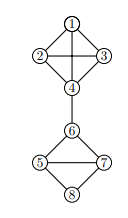# Let G be a graph whose vertices are the integers 1 through 8, and let the adjacent vertices of...

## Question:

Let G be a graph whose vertices are the integers 1 through 8, and let the adjacent vertices of each vertex be given by the table below.

1 (2,3,4)
2 (1,3,4)
3 (1,2,4)
4 (1,2,3,6)
5 (6,7,8)
6 (4,5,7)
7 (5,6,8)
8 (5,7)

Assume that, in a traversal of G, the adjacent vertices of a given vertex are returned in the same order as they are listed in the above table.

a. Draw G.

b. Order the vertices as they are visited in a DFS traversal starting at vertex 1.

c. Order the vertices as they are visited in a BFS traversal starting at vertex 1.

## Searching Graphs:

The two ways to search the nodes of graphs are Depth First Search (DFS) and Breadth First Search (BFS). DFS dives as deep as it can to children of children before backtracking at each dead end. BFS looks across each level, then the next level of children.

a. Draw G.b. Give the sequence of vertices of G visited using a DFS traversal starting at vertex 1.

Depth First Search visits all the...

Become a Study.com member to unlock this answer! Create your account